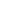DASCA is the World’s Foremost Standards & Credentialing Body for the Data Science Profession.

Identifying and Removing Outliers Using Python Packages

# Insights

## Identifying and Removing Outliers Using Python PackagesValidity, accuracy in interpretation and applicability in business contexts are critical fundamentals to the overall insights that form the essence of Exploratory Data Analysis (EDA) in any machine learning projects. In the entire EDA process, the anomaly that outliers cause are often a source of frustration for data scientists and machine learning engineers. Especially prominent in the case of data visualization projects and statistical models, taking away from the objectivity of the project at hand.

OUTLIERS

Observations in statistics that are far removed from the normalized distribution observation in any data set in statistics form the gist of outliers. The most common reasons that outliers occur include an error in measurement or input of the data, corrupt data, and the typical true observation that’s outside the normal distribution. Because of the very nature of datasets in data science, a mathematical definition of an outlier cannot really be defined specifically. However, close observation of the dataset with some prior knowledge is required to accurately identify outliers.

As mentioned above, machine learning algorithms and general data visualization projects are drastically affected when outliers are overlooked due to errors of omission or being far from the normal statistical distribution in a dataset.

IDENTIFYING OUTLIERS

There are several methods that data scientists employ to identify outliers. The ends drive the means, in this case. To exemplify, pattern differentials in a scatter plot is by far the most common method in identifying an outlier.

Using Z score is another common method. Basically defined as the number of standard deviations that the data point is away from the mean. Also known as standard scores, Z scores can range anywhere between -3 standard deviations to +3 standard deviations on either side of the mean. It’s usually calculated as z = (x-μ) ̸ σ. Z-score has its limitations, though, and there are variations of this method to identify outliers in multiple datasets as well as include certain modifiers for better accuracy.

Another method is the Inter Quartile Range, also referred to as IQR, is the difference between the fourth and three fourth percentiles – aka the upper and lower quartiles of a dataset.

THE BASICS OF QUANTILES

Quantiles essentially refer to the mathematical expressions of the borderlines of each segment within the dataset. The nomenclature is fairly common and easy to understand, with percentile referring to a 100, decile referring to 10 and quartile referring to 4. Quantiles, in this case, refer to n where n is the number of segments in the dataset.

As a natural consequence, the interquartile range of the dataset would ideally follow a breakup point of 25%.

With that understood, the IQR usually identifies outliers with their deviations when expressed in a box plot. Observations below Q1- 1.5 IQR, or those above Q3 + 1.5IQR (note that the sum of the IQR is always 4) are defined as outliers.

USING NUMPY

For Python users, NumPy is the most commonly used Python package for identifying outliers. If you’ve understood the concepts of IQR in outlier detection, this becomes a cakewalk. For a dataset already imported in a python instance, the code for installing NumPy and running it on the dataset is:

```import numpy as np
def removeOutliers(x, outlierConstant):
a = np.array(x)
upper_quartile = np.percentile(a, 75)
lower_quartile = np.percentile(a, 25)
IQR = (upper_quartile - lower_quartile) * outlierConstant
quartileSet = (lower_quartile - IQR, upper_quartile + IQR)
resultList = []
for y in a.tolist():
if y > = quartileSet and y < = quartileSet:
resultList.append(y)
return resultList

```

(Source: Github)

The results returned above would be the outliers.

USING PANDAS

Pandas is another hugely popular package for removing outliers in Python. In the code snippet below, numpy and pandas are used in tandem to remove outliers in the name, age and address variables in a dataset:

```import pandas as pd
import numpy as np
from pandas.api.types import is_numeric_dtype
np.random.seed(42)
age = np.random.randint(20,100,50)
name = ['name'+str(i) for i in range(50)]
def remove_outlier(df):
low = .05
high = .95
quant_df = df.quantile([low, high])
for name in list(df.columns):
if is_numeric_dtype(df[name]):
df = df[(df[name] > quant_df.loc[low, name])
& (df[name] < quant_df.loc[high, name])]
return df
```

(Source: Github)

CAVEATS

While outlier removal forms an essential part of a dataset normalization, it’s important to ensure zero errors in the assumptions that influence outlier removal. Data with even significant number of outliers may not always be bad data and a rigorous investigation of the dataset in itself is often warranted, but overlooked, by data scientists in their processes.

EDA is one of the most crucial aspects in any data science projects, and an absolutely must-have before commencement of any machine learning projects. Achieving a high degree of certainty and accuracy on the validity, interpretation and applicability of the data set and the project in general ensures desired business outcomes.

## Suggested Articles

> <
X

This website uses cookies to enhance website functionalities and improve your online experience. By browsing this website, you agree to the use of cookies as outlined in our privacy policy.

Got it### How to Register for Your DASCA CertificationWatch Now!